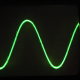## Sensors, Electronics and Prototyping# Reply To: Understand checksum value

Welcome to Redshift Labs Forums UM7 Product Support Understand checksum value Reply To: Understand checksum value

#1185till
Guest

hi matteo,

Please see UM7 Datasheet page 37:

``````uint16_t computed_checksum = 's' + 'n' + 'p' + packet_data->PT + packet_data->Address;
for( index = 0; index < data_length; index++ ) {
packet->data[index] = rx_data[packet_index + 5 + index];
computed_checksum += packet->data[index];
}
uint16_t received_checksum = (rx_data[packet_index + 5 + data_length] << 8);
received_checksum |= rx_data[packet_index + 6 + data_length];
``````

checksum1 and checksum0 are just the two bytes making up the 16-bit short integer. you take a 16-bit integer initialized to 0 and then add the value of each byte in the packet to calculate the checksum.

the received checksum consists of two bytes which you have to combine to a 16-bit integer.

regards,

-till